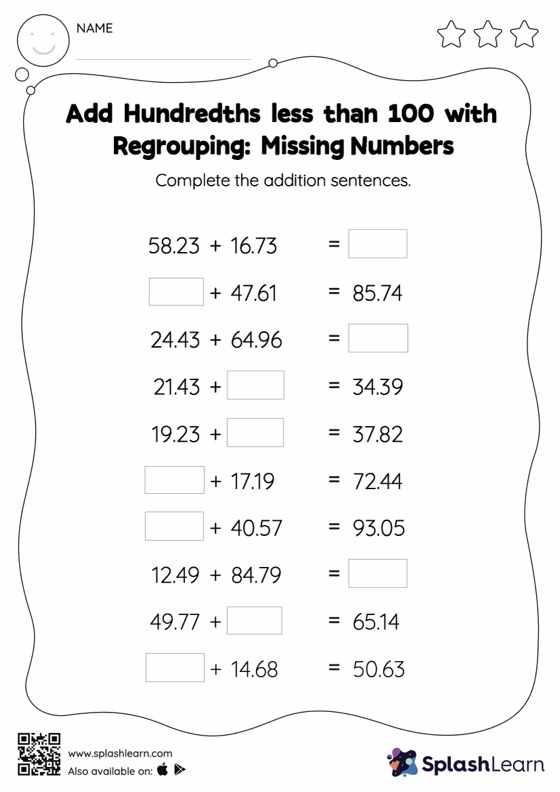# Add Hundredths less than 100 with Regrouping: Missing Numbers Worksheet

Home > Add Hundredths less than 100 with Regrouping: Missing NumbersTask your little mathematicians to crack the code of adding hundredths less than 100 with regrouping with this fun worksheet. While adding decimals, students use the relationship between addition and subtraction to find the missing number. They also need to regroup numbers in add hundredths less than 100 with regrouping worksheet utilizing the relationship between ones, tenth, hundredth, thousandth, etc., To get to the result. In each problem, the numbers are laid out in the horizontal format. Students should try to use different strategies involving composing and decomposing numbers to solve these problems. This will help them develop flexibility and fluency.